# Fitting a basic Fay-Herriot model in Stan and JAGS

A short post showing how to fit a very basic, Bayesian Fay-Herriot model in Stan and JAGS. This post focuses on model specification, fitting, and visualization.

Ben Schneider
5-1-2022

## Overview

This post builds on some of what I learned from the Small Area Estimation course at JPSM as well as a very interesting small area estimation project for work, which resulted in the recently-published paper with Westat colleagues Andreea Erciulescu and Jean Opsomer:

For that project, we developed a model which is essentially a multivariate extension of the Fay-Herriot model, where some domains have unobserved values for one or more outcome variables. We first fit the model in JAGS, but we also tried using Stan in order to experiment with non-conjugate prior distributions for the variance components of the model. In this post, I share some of what I learned about fitting Bayesian Fay-Herriot models in JAGS and Stan, using publicly-available wage data from BLS for the purpose of illustration.

## Background

The Fay-Herriot model is perhaps the most fundamental model in the field of small area estimation (SAE). The basic idea is simple. Suppose you want to estimate some quantity, such as the average wage, for each of several different cities, and you have only limited data from each city. To estimate an average wage for a given city in a given region, we have a couple of obvious options:

• The “direct estimate” approach. Produce a noisy estimate using just that city’s data. If the underlying sample data are representative and appropriate statistical procedures are used, then the estimates are unbiased but imprecise.

• The “completely pooled estimate”. Simply produce a single pooled estimate by aggregating together all of the estimates from cities in the region. Then use that as the estimate for each city in the region. Obviously this estimate is biased for each city (since cities should differ from one another), but the estimate is very precise and so might be better than the noisy estimate based on only the city’s data.

The Fay-Herriot model allows a compromise approach: for each city, use a weighted average of the city’s direct estimate and the regional average. For cities where the direct estimate is relatively precise (for example, if the sample size is large), assign more weight to the city’s direct estimate and less weight to the completely pooled region estimate. And in general, assign more weight to cities’ direct estimates if there appears to be large variation between cities. This strategy is referred to as “partial pooling”: estimates for a specific city are based on pooling together data from the specific city as well as other cities, but the city’s data is given more weight if it seems reliable.

In principle, this is an intuitive idea that probably feels like it’s just common sense. This is just a statistical way of saying the following:

“Consider the context. If a city’s noisy estimate is way higher than estimates from other similar cities, it’s probably an overestimate and we should adjust it to look more like the average city’s estimate.”

Of course, the devil is in the details.

## Example data

For this example, we’ll use wage data published by the Bureau of Labor Statistics (BLS) as part of its Occupational Employment and Wage Statistics (OEWS) program. These data consist of estimated total employment and average wages in 2020 for hundreds of occupations in hundreds of metropolitan statistical areas (MSAs). Each estimate is accompanied with a standard error. 1

# Download the '.zip' file from BLS and extract the Excel file
temp_zip_file <- tempfile(fileext = ".zip")
temp_data_folder <- tempdir()

unzip(zipfile = temp_zip_file,
exdir = temp_data_folder,
files = "oesm20ma/MSA_M2020_dl.xlsx")

# Read in the data from the Excel file
"MSA_M2020_dl.xlsx"),
sheet = "MSA_M2020_dl")


Show data processing code
library(dplyr)

msa_data <- raw_msa_data %>%
select(
# MSA name and code
AREA, AREA_TITLE,
# Occupation name and code
O_GROUP, OCC_CODE, OCC_TITLE,
# Employment estimate, and its std. error
TOT_EMP, EMP_PRSE,
# Mean annual wage estimate, and its std. error
A_MEAN_WAGE = A_MEAN, A_MEAN_WAGE_PRSE = MEAN_PRSE
) %>%
# Ensure variables are stored as numeric
# and standard errors are on the same scale as the estimate
mutate(across(matches("EMP|MEAN"), as.numeric)) %>%
mutate(TOT_EMP_SE = (EMP_PRSE/100) * TOT_EMP,
A_MEAN_WAGE_SE = (A_MEAN_WAGE_PRSE/100) * A_MEAN_WAGE) %>%
select(-EMP_PRSE, -A_MEAN_WAGE_PRSE) %>%
# Rearrange columns for ease of reading
select(matches("AREA"), starts_with("O"),
starts_with("TOT_EMP"), starts_with("A_MEAN_WAGE"))


AREA AREA_TITLE O_GROUP OCC_CODE OCC_TITLE TOT_EMP TOT_EMP_SE A_MEAN_WAGE A_MEAN_WAGE_SE
10180 Abilene, TX total 00-0000 All Occupations 66060 1255.14 42930 772.74
10180 Abilene, TX major 11-0000 Management Occupations 2910 130.95 89160 1961.52
10180 Abilene, TX detailed 11-1021 General and Operations Managers 1320 97.68 83990 2939.65
10180 Abilene, TX detailed 11-2022 Sales Managers 90 16.65 121020 9197.52
10180 Abilene, TX detailed 11-2030 Public Relations and Fundraising Managers 40 12.60 95540 19776.78

For context, the estimates are available for occupations within metropolitan statistical areas (MSAs), and occupations are defined at both a detailed level (a six-digit code known as an SOC6) and a major level (a two-digit code known as an SOC2), where detailed SOC6 occupations are nested within major SOC2 occupations. This suggests that if we’re interested in estimates for detailed occupations in MSAs, we can improve our estimates by pooling across MSAs within a region and/or by pooling across detailed SOC6 occupations within a major SOC2 occupation.

## Inspect the data

Let’s take a look at the distribution of wage estimates across MSAs, within each major occupation group. We’ll focus on the MSAs where the estimates appear to be relatively precise (i.e. where the estimated percent relative standard error is under 10%); this will allow us to get a sense of what the actual variability across MSAs looks like. At a first glance, it looks like a log-normal distribution would provide a reasonable model of variability across MSAs.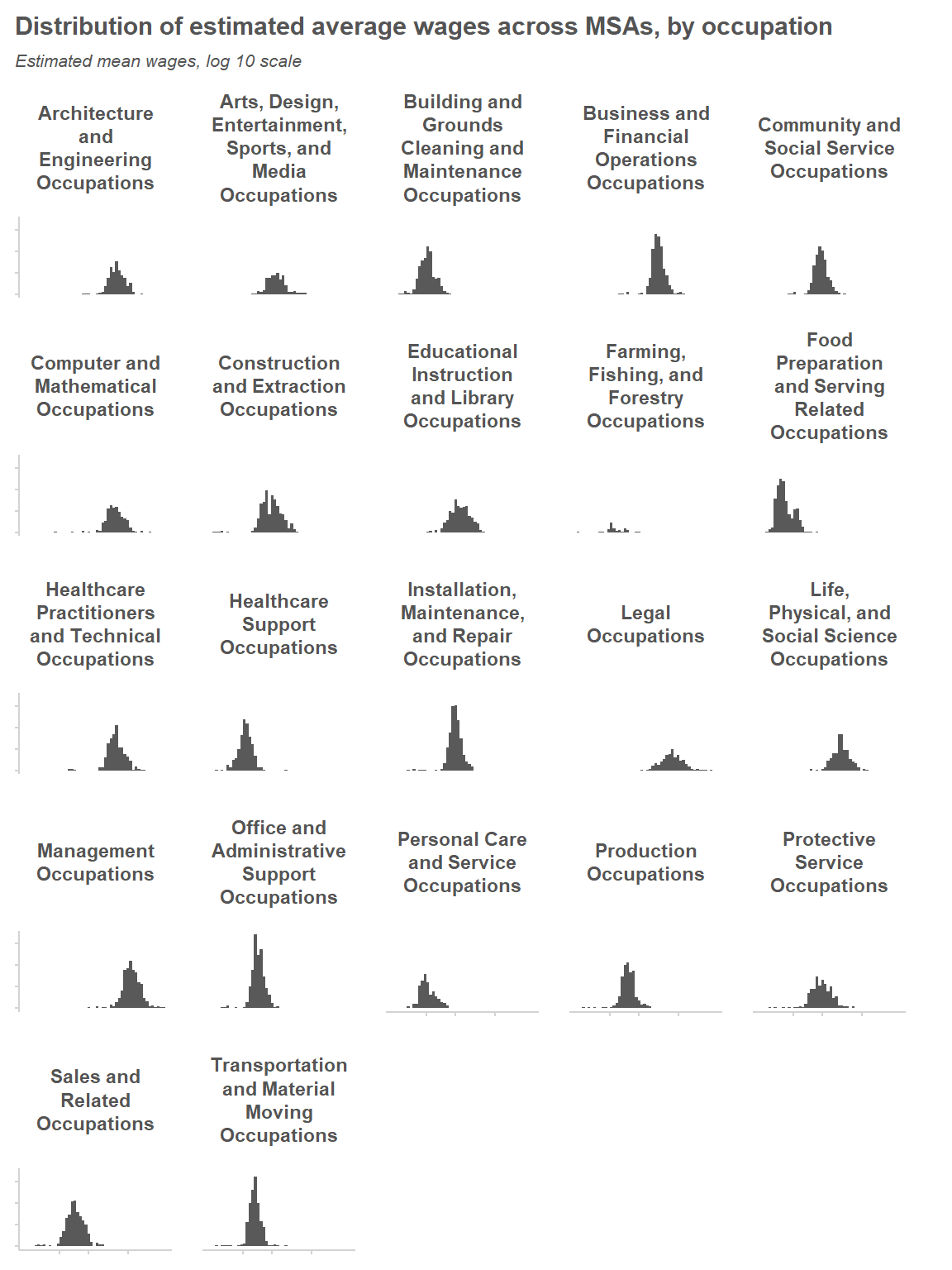Next we’ll try to see how (im)precise the detailed occupational wage estimates are.

AREA_TITLE MAJOR_OCC OCC_TITLE A_MEAN_WAGE A_MEAN_WAGE_SE
Abilene, TX Management Occupations General and Operations Managers 83990 2939.65
Abilene, TX Management Occupations Sales Managers 121020 9197.52

From the plot, it is apparent that the PRSEs are all below 30%. This reflects BLS publication standards preventing the publication of highly imprecise estimates; estimates whose PRSE are above 30% are generally not published. The 90% quantiles for the PRSEs are generally between 8% and 14%.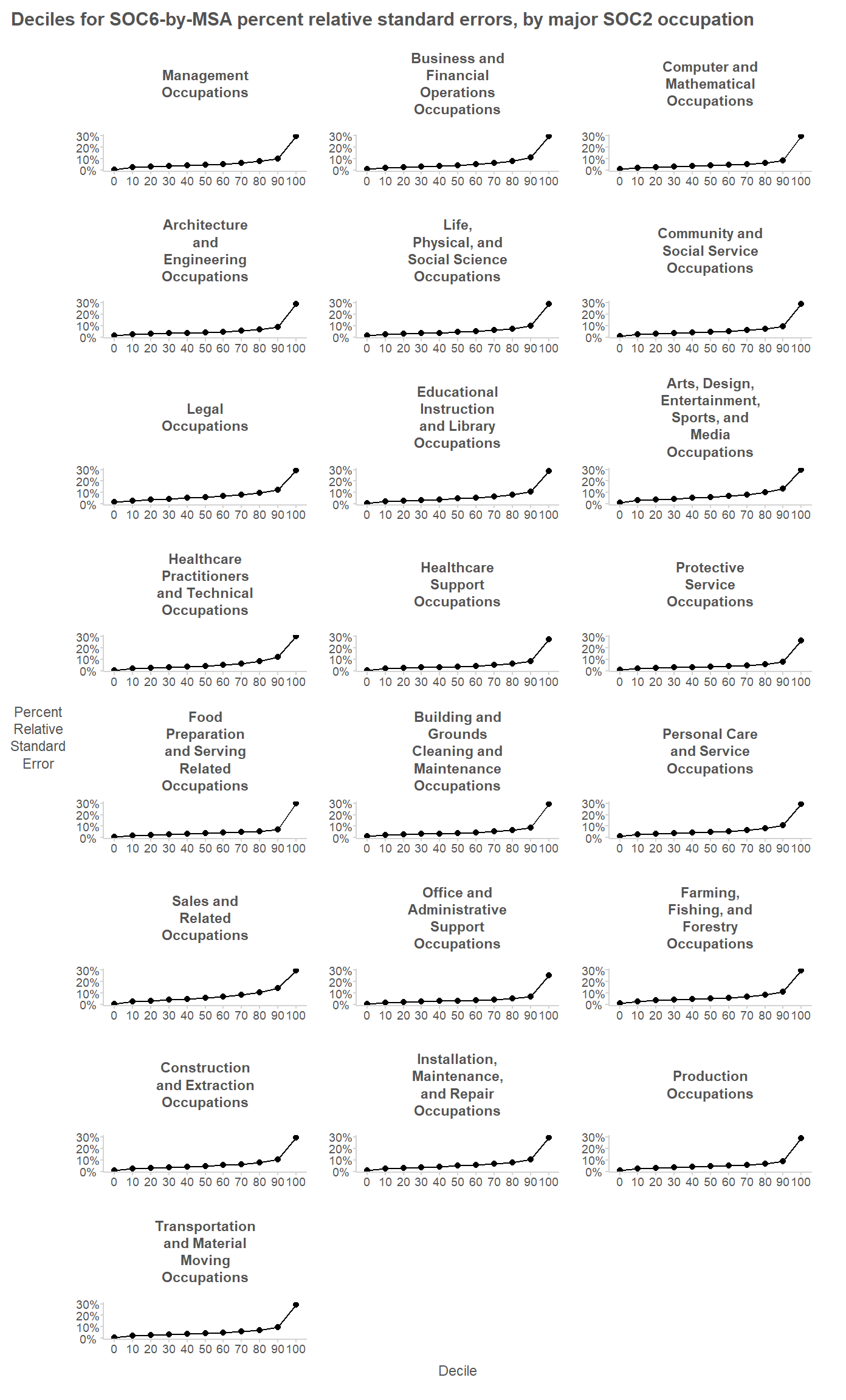## Fit a basic model

For both Stan and JAGS, we have to write our model using special language. This written model can be passed to Stan/JAGS either as a string in R (e.g. "y ~ normal(0, 1)") or by supplying a standalone file saved on your computer. Below we’ll write out our model in mathematical notation and in the special modeling languages for Stan and for JAGS.

The average annual wage for a given domain (i.e. a given occupation in a given city) is denoted $$\theta_j$$. The direct estimate based on survey data from just that domain is $$\hat{\theta}_j$$, and its sampling variance is $$V_j$$.

Because the variation in average wages across domains seems to follow a log-normal distribution, we’ll model the log-transformed parameter, $$\theta_{j,log}=ln(\theta_j)$$ and its estimate $$\hat{\theta}_{j,log}=log(\hat{\theta_j})$$. To obtain the sampling variance of $$\hat{\theta}_{j,log}=log(\hat{\theta_j})$$, we’ll use the Delta Method: $$V_{j,log} := \frac{1}{(\hat{\theta})^2}V_j$$. Note that $$V_{j,log}$$ is an estimate (and so is $$V_j$$), but for modeling purposes we ignore that fact and assume that these are known values. This assumption can be relaxed but we’ll avoid getting into the complicated variance modeling required to do so.

\begin{aligned} \\ \underline{\textbf{Sampling }\textbf{Level}}& \\ \textbf{Likelihood}& \\ \hat{\theta}_{j,log} &\sim {N}\left(\theta_{j,log},V_{j,log}\right) \\ \underline{\textbf{Smoothing }\textbf{Level}}& \\ \textbf{Likelihood}& \\ \theta_{j,log} &\sim {N}\left(\mu,\sigma^2\right) \\ \textbf{Priors}& \\ \mu &\sim {N}\left(\ln(50,000), \ln(50,000)^2 \space\right) \\ \sigma^2 &\sim \text{Inv-Gamma}\left(0.001, 0.001\right) \end{aligned}

Stan Model Syntax

data {
int<lower=0> J;
real dir_est[J];
real<lower=0> dir_est_std_error[J];
}
parameters {
real theta[J];
real mu;
real<lower=0> sigma2;
}
model {
// Likelihood
//-- Sampling level
dir_est ~ normal(theta, dir_est_std_error) ;
//-- Smoothing level
theta ~ normal(mu, sqrt(sigma2)) ;
// Priors
//-- Uninformative prior on variance of distribution
sigma2 ~ inv_gamma(0.001, 0.001);
//-- Weakly informative prior on center of distribution
mu ~ normal(log(50000),log(50000))
}

JAGS Model Syntax

model {
# Likelihood
for (j in 1:J) {
dir_est_precision[j] <- 1/dir_est_std_error[j]^2
dir_est[j] ~ dnorm(theta[j], dir_est_precision[j])
theta[j] ~ dnorm(mu, inv_sigma2)
}
# Priors
## Uninformative prior on variance of distribution
inv_sigma2 ~ dgamma(0.001, 0.001)
sigma2 <- 1/inv_sigma2
## Weakly informative prior on center of distribution
mu ~ dnorm(log(50000), log(50000))
}

How were those priors chosen? For the prior on $$\sigma^2$$, I used a common uninformative prior for the variance of a Normal distribution. The Inverse-Gamma distribution is a conjugate prior for this case, and so it can be used in both Stan and JAGS.

For the prior on $$\mu$$, I wanted a weakly informative prior and so I chose a Normal prior that, on the non-logarithmic scale, is centered at right about the average annual wage for the U.S. overall, and which is diffuse in the sense that it has a coefficient of variation of 100%. The reason for using a weakly-informative prior in this case is that we do have good external information about the center of the wage distribution which we can easily incorporate into our model, and doing so may slightly improve the precision of our estimates.

### Using JAGS

We’ll fit this basic model in JAGS first. The input data needs to be an R list object, with elements whose names match the data described in our JAGS model.

# Prepare the data for JAGS ----
model_data <- msa_by_soc6_data[,c("AREA_TITLE", "OCC_TITLE",
"A_MEAN_WAGE", "A_MEAN_WAGE_SE")] %>%
na.omit()

model_input_data <- list(
J = nrow(model_data),
dir_est = log(model_data$A_MEAN_WAGE), dir_est_std_error = (1/model_data$A_MEAN_WAGE) * model_data$A_MEAN_WAGE_SE ) # Prepare the model for MCMC sampling ---- jags_fh_model <- rjags::jags.model( data = model_input_data, file = textConnection( "model { # Likelihood for (j in 1:J) { dir_est_precision[j] <- 1/dir_est_std_error[j]^2 dir_est[j] ~ dnorm(theta[j], dir_est_precision[j]) theta[j] ~ dnorm(mu, inv_sigma2) } # Priors ## Uninformative prior on variance of distribution inv_sigma2 ~ dgamma(0.001, 0.001) sigma2 <- 1/inv_sigma2 ## Weakly informative prior on center of distribution mu ~ dnorm(log(50000), log(50000)) }" ), n.chains = 2 )  Compiling model graph Resolving undeclared variables Allocating nodes Graph information: Observed stochastic nodes: 127833 Unobserved stochastic nodes: 127835 Total graph size: 384096 Initializing model After declaring the model, we use the update() function to “burn-in” a desired number of iterations. update(jags_fh_model, n.iter = 1000)  Then we can use the code.samples() function to draw a sample with a desired thinning rate. By using the variable.names argument, we can control which variables to retain in our output of posterior draws; this can be useful if there are many nuisance parameters. A Note on Thinning While thinning is counterproductive in many (if not most) cases, it is in fact helpful here. Despite the popular misconception that thinning is necessary for MCMC inference, the decision to use a thinned chain almost always worsens the quality of our estimates compared to using an unthinned chain (see Link and Eaton 2010), as we are reducing our Monte Carlo sample size. However, thinning increases the information content of data of a given size. So when our model is large (as in our example, with its 100,000+ parameters of interest) and our ability to store the posterior draws and work with them is limited by memory/computational constraints, thinning gets us more value from our costly storage and computations. # Draw MCMC samples ---- ## Run the chain for 5,000 iterations, ## retaining every 10th iteration jags_posterior_samples <- coda.samples( model = jags_fh_model, n.iter = 5000, thin = 10, variable.names = c('theta', 'mu', 'sigma2') )  Let’s examine the output from the samples. We can obtain a neat data frame of results using the tidy_draws() function from the handy {tidybayes} R package: one row per draw per chain, and one column per parameter of interest. tidy_jags_samples <- tidybayes::tidy_draws(model = jags_posterior_samples) str(tidy_jags_samples[,1:10])  tibble [1,000 × 10] (S3: tbl_df/tbl/data.frame)$ .chain    : int [1:1000] 1 1 1 1 1 1 1 1 1 1 ...
$.iteration: int [1:1000] 1 2 3 4 5 6 7 8 9 10 ...$ .draw     : int [1:1000] 1 2 3 4 5 6 7 8 9 10 ...
$mu : num [1:1000] 10.8 10.8 10.8 10.8 10.8 ...$ sigma2    : num [1:1000] 0.22 0.219 0.22 0.219 0.221 ...
$theta : num [1:1000] 11.3 11.3 11.4 11.3 11.3 ...$ theta  : num [1:1000] 11.6 11.7 11.8 11.8 11.6 ...
$theta : num [1:1000] 11.1 11.4 11.3 11.3 11.1 ...$ theta  : num [1:1000] 11.2 11.3 11.3 11.2 11.2 ...
$theta : num [1:1000] 11.7 11.8 11.7 11.7 11.8 ... To summarize the posterior draws for a given parameter, we simply work with the rows for a column of interest. For illustration, we’ll look at the posterior distribution for average wages among statisticians in D.C. # Determine name of the parameter ---- j_dc_statistician <- model_data %>% mutate(j = row_number()) %>% filter(stringr::str_detect(OCC_TITLE, "Statistician"), stringr::str_detect(AREA_TITLE, "Washington.+DC")) %>% pull("j") print(j_dc_statistician)   115355 param_of_interest <- sprintf("theta[%s]", j_dc_statistician) print(param_of_interest)   "theta" # Extract posterior draws and exponentiate # (since the model is specified on the log scale) param_samples <- exp(tidy_jags_samples[[param_of_interest]]) # Calculate summaries of interest (mean and standard deviation) post_mean <- mean(param_samples) post_sd <- sd(param_samples) # Format summaries into text text_summary <- sprintf( "Mean: %s\nStandard Deviation: %s", mean(exp(tidy_jags_samples[[param_of_interest]])) %>% scales::dollar(), sd(exp(tidy_jags_samples[[param_of_interest]])) %>% scales::comma() ) writeLines(text_summary)  Mean:$113,213
Standard Deviation: 3,196

To visualize the posterior sample, we can use the handy mcmc_areas() function from the {bayesplot} package.

library(bayesplot)

mcmc_areas(jags_posterior_samples,
pars = param_of_interest,
transformations = "exp", prob = 0.95) +
scale_x_continuous(labels = scales::dollar) +
labs(
title = "Posterior Distribution of Mean Wages for Statisticians in DC",
x = "Average Wage",
y = "Posterior\nDensity",
subtitle = text_summary
)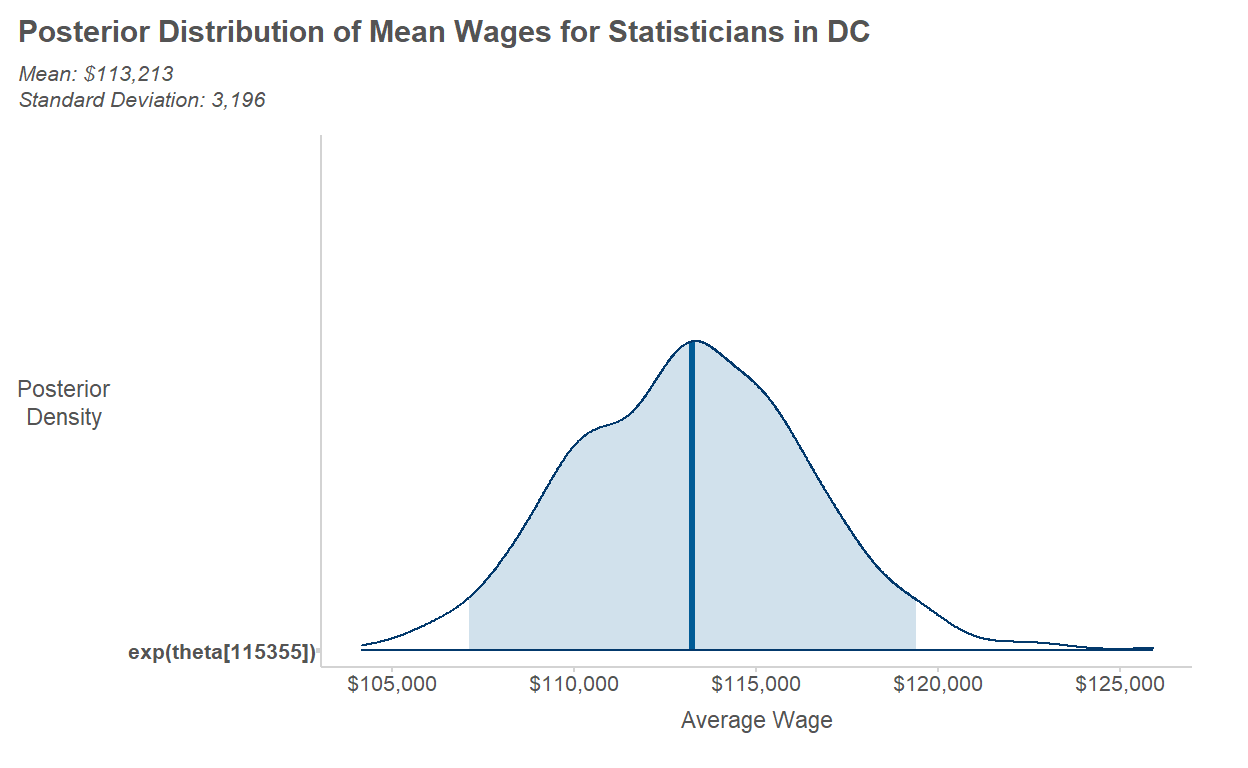### Using Stan

Now let’s fit the same model using Stan. There are a couple of different packages we can use–{rstan} or the in-development {cmdstanr}–but here we’ll stick with {rstan} because it’s more stable and better documented.

First, we write out our model in Stan code, either as a ‘.stan’ file or as a character string. Because our model is fairly small, we’ll use a character string for the sake of illustration. Then we supply the model and our data to the function stan(). Note that we can use the exact same input data for Stan here as we used for JAGS.

model_code <-
"data {
int<lower=0> J;
real dir_est[J];
real<lower=0> dir_est_std_error[J];
}
parameters {
real theta[J];
real mu;
real<lower=0> sigma2;
}
model {
// Likelihood
//-- Sampling level
dir_est ~ normal(theta, dir_est_std_error) ;
//-- Smoothing level
theta ~ normal(mu, sqrt(sigma2)) ;
// Priors
//-- Uninformative prior on variance of distribution
sigma2 ~ inv_gamma(0.001, 0.001);
//-- Weakly informative prior on center of distribution
mu ~ normal(log(50000),log(50000));
}"

library(rstan)

stan_fit <- stan(
model_code = model_code,  # Stan program
data = model_input_data,  # named list of data
chains = 2,               # number of Markov chains
warmup = 1000,            # number of warmup iterations per chain
iter = 6000,              # total number of iterations per chain (incl. warmup)
thin = 10,                # thinning interval
cores = 2,                # number of cores (could use one per chain)
refresh = 500             # refresh progress bar every 500 iterations
)


This model took much longer to fit in Stan than in JAGS (10 minutes versus two hours). For the same amount of computational time required to use Stan here, we could have used JAGS to run more chains for more iterations. However, some benefits of the Stan model include more built-in MCMC diagnostics and, if we had chosen, better ability to handle non-conjugate prior distributions.

Just like we did with the JAGS output, we can use the {tidybayes} package to obtain a tidy data frame of the posterior draws.

tidy_stan_samples <- tidybayes::tidy_draws(model = stan_fit)
str(tidy_stan_samples[,1:10])

tibble [800 × 10] (S3: tbl_df/tbl/data.frame)
$.chain : int [1:800] 1 1 1 1 1 1 1 1 1 1 ...$ .iteration: int [1:800] 1 2 3 4 5 6 7 8 9 10 ...
$.draw : int [1:800] 1 2 3 4 5 6 7 8 9 10 ...$ theta  : num [1:800] 11.3 11.3 11.3 11.3 11.4 ...
$theta : num [1:800] 11.6 11.7 11.8 11.7 11.7 ...$ theta  : num [1:800] 11.1 11.1 11.3 11 11.4 ...
$theta : num [1:800] 11.3 11.3 11.3 11.3 11.2 ...$ theta  : num [1:800] 11.8 11.6 11.6 11.7 11.7 ...
$theta : num [1:800] 11.7 11.6 11.6 11.7 11.7 ...$ theta  : num [1:800] 11.7 11.4 11.6 11.5 11.7 ...

## Comparing Stan and JAGS Fits

With the tidied outputs in hand, we can compare the posterior estimates from Stan and JAGS. First, we’ll create a data frame with the original estimates side-by-side with the posterior means and standard deviations from both of the two pieces of software.

# A tibble: 6 × 8
AREA_TITLE  OCC_TITLE      A_MEAN_WAGE A_MEAN_WAGE_SE POST_MEAN_STAN
<chr>       <chr>                <dbl>          <dbl>          <dbl>
1 Abilene, TX General and O…       83990          2940.         83886.
2 Abilene, TX Sales Managers      121020          9198.        118865.
3 Abilene, TX Public Relati…       95540         19777.         86303.
4 Abilene, TX Administrativ…       78600          2751          78416.
5 Abilene, TX Computer and …      123040         10458.        119463.
6 Abilene, TX Financial Man…      119580         11480.        115419.
# … with 3 more variables: POST_MEAN_JAGS <dbl>, POST_SD_STAN <dbl>,
#   POST_SD_JAGS <dbl>

Let’s see whether the Stan and JAGS estimates are similar (as we’d hope). The correlation is nearly 1.0 and, the relative difference in the domain estimates rarely exceeds 1% and never exceeds 4%.

 0.9999732
 0.9988437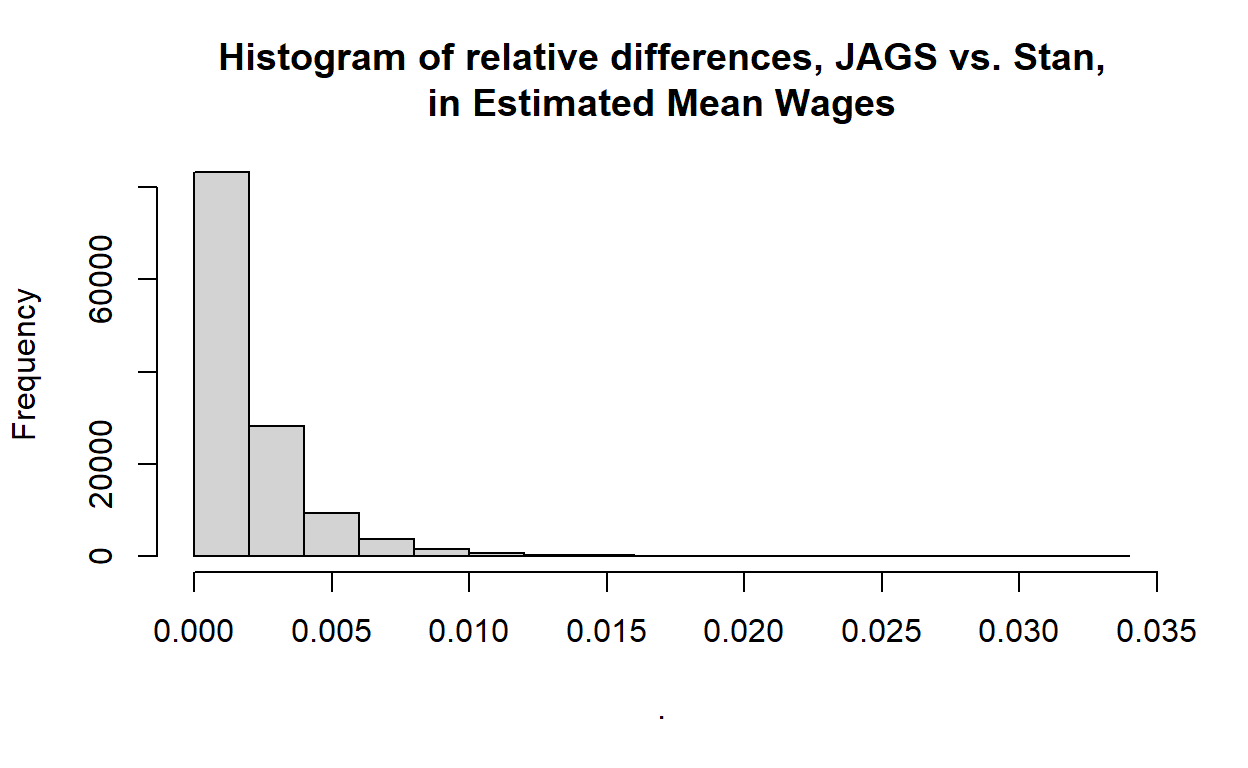In short, the estimates from fitting this model in Stan vs. JAGS are essentially the same. But there are some small discrepancies likely attributable to random variation that occurs in any MCMC simulation, and so we would want to increase the number of draws if we were actually going to publish or otherwise act upon these estimates.

## Evaluating the Pooling Induced by the Fay-Herriot Model

Next we can plot the original direct estimates against the estimates from the Fay-Herriot model. For the sake of illustration, we can highlight one particular domain where there is a large difference between the original direct estimate and the posterior mean from the Fay-Herriot model.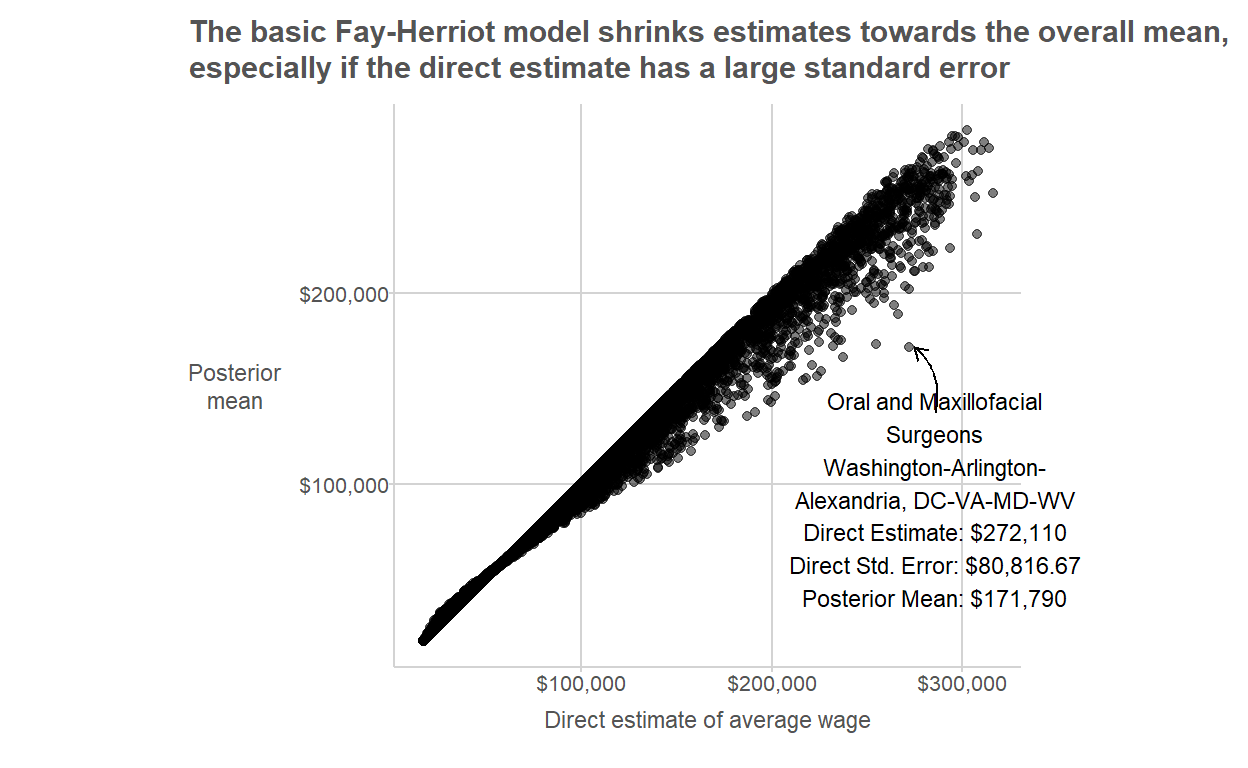## Some Potential Improvements for the Model

This basic model has a couple of obvious issues. For starters, it ignores big, systematic patterns of variation in the data. Wages vary dramatically across regions and across major SOC2 groups. For example, the average wage in the D.C. metro area is about \$10/hour higher than in Miami, and occupations in the “Food Preparation and Serving” SOC2 group usually have lower wages than occupations in the “Healthcare Practitioners and Technical Occupations” SOC2 group. But when the model produces estimates for oral surgeons in the Washington D.C. MSA, it is just as influenced by data from waiters in Miami as by data from nurses in Virginia or Maryland. This results in model-induced mistakes such as that illustrated in the plot below, which shows that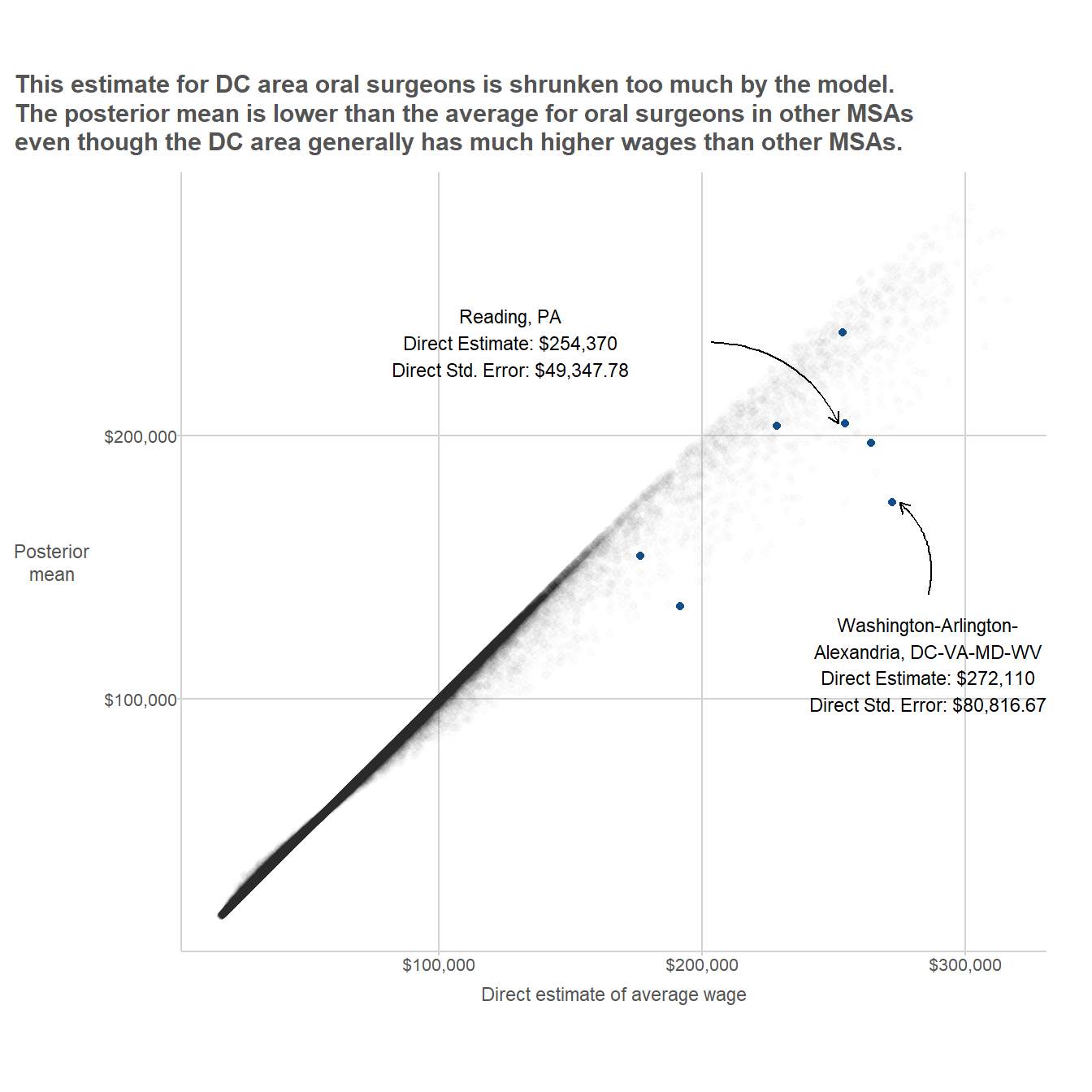A better model would make use of the fact that when estimating wages for oral surgeons, the data on nurses in the same state are more relevant than data from non-medical professions in other states. One way to do this would be to add random effects for geographic areas (MSAs, states, or some combination thereof) and occupations (at the SOC2 or perhaps SOC6 level). That’s the approach that Andreea, Jean, and I took in the paper mentioned at the beginning of this post.

1. The data file represents standard errors as a “percent relative standard error (PRSE)”, which is the ratio of an estimate’s standard error to the estimate itself, expressed as a percentage.↩︎

2. There are actually multiple fine R packages for downloading BLS data, I just don’t have the motivation to figure out which one to use or how to use it.↩︎

### Citation

Schneider (2022, May 1). Practical Significance: Fitting a basic Fay-Herriot model in Stan and JAGS. Retrieved from https://www.practicalsignificance.com/posts/basic-fay-herriot-code/
@misc{schneider2022fitting,
}